Courses

# Tacheometric, Curve, Hydrographic and Plane Table Surveying & Theory of Errors Civil Engineering (CE) Notes | EduRev

## Civil Engineering (CE) : Tacheometric, Curve, Hydrographic and Plane Table Surveying & Theory of Errors Civil Engineering (CE) Notes | EduRev

The document Tacheometric, Curve, Hydrographic and Plane Table Surveying & Theory of Errors Civil Engineering (CE) Notes | EduRev is a part of the Civil Engineering (CE) Course Topic wise GATE Past Year Papers for Civil Engineering.
All you need of Civil Engineering (CE) at this link: Civil Engineering (CE)

Question 1: The method of orientation used, when the plane table occupies a position not yet located on the map, is called as    [2017 : 1 Mark, Set-II]
(a) traversing
(b) radiation
(c) levelling
(d) resection

Answer: (d)
Solution: 1. Traversing: 'Traverse’ means to pass across. Traversing refer to the framework of series of lines forming an open or closed polygon.
2. Radiation: It is a method of locating a point by drawing a radial line from the plane table station to the station under consideration.
3. Levelling: It is the method of determining the difference of elevations or levels of different points on the earth’s surface.
4. Resection: It is the method of locating a station occupied by the plane table when the position of that station has not been plotted earlier on the sheet when the plane table occupied other station

Question 2: The VPI (vertical point of intersection) is 100 m away (when measured the horizontal) from the VPC (vertical point of curvature). If the vertical curve parabolic, the length of the curve (in meters and measured along the horizontal) is ________.    [2017 :1 Mark, Set-II]
Solution: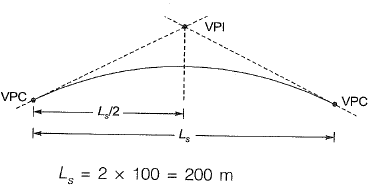Question 3: A circular curve of radius R connects two straights with a deflection angle of 60°. The tangent length is    [2016 : 1 Mark, Set-II]
(a) 0.577 R
(b) 1.155 R
(c) 1.732 R
(d) 3.464 R
Answer: (a)
Solution: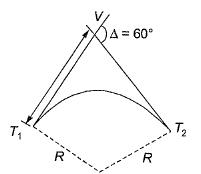Tangent length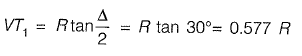Question 4: In a survey work, three independent angles, X, Y and Z were observed with weights Wx, WY, Wz, respectively. The weight of the sum of angles X, Y and Z is given by    [2015 : 2 Marks, Set-T]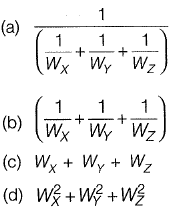Answer: (a)
Solution: Various laws of weight
Let there be quantities A and B with weight Wa and Wb respectively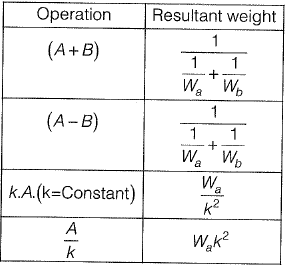Question 5: The chainage of the intersection point of two straights is 1585.60 m and the angle of intersection is 140°. If the radius of a circular curve is 600.00 m, the tangent distance (in m) and length of the curve (in m), respectively are    [2014 : 2 Marks, Set-II]
(a) 418.88 and 1466.08
(b) 218.38 and 1648.49
(c) 218.88 and 418.82
(d) 418.88 and 218.38
Answer: (c)
Solution: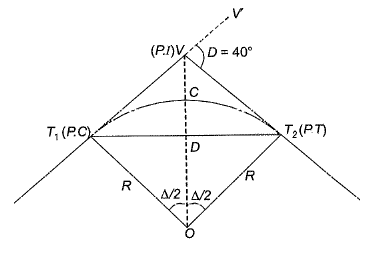Δ = 180° - 140° = 40°
Length of the curve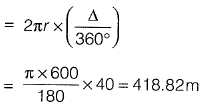Tangent distance (7) is the distance between
P-C to P.I (also the distance from P.I to P. T)
⇒ T = T1V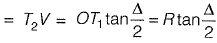= 600 tan 20° = 218.88 m

Question 5: A tacheometer was placed at point P to estimate the horizontal distances PQ and PR. The corresponding stadia intercepts with the telescope kept horizontal, are 0.320 m and 0.210 m, respectively. The ∠QPR is measured to be 61° 30' 30". If the stadia multiplication constant = 100 and stadia addition constant = 0.10 m, the horizontal distance (in m) between the points Q and R is ______.     [2014 : 2 Marks, Set-II]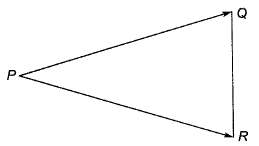Answer: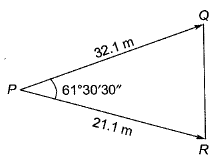PQ = ks + C
= 100(0.32) + 0.1 = 32.1 m
PR = ks + C
= 100(0.21) + 0.1 = 21.1 m
Applying the cosine rule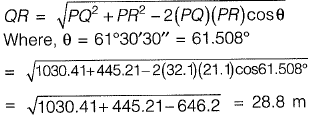Offer running on EduRev: Apply code STAYHOME200 to get INR 200 off on our premium plan EduRev Infinity!

69 docs

,

,

,

,

,

,

,

,

,

,

,

,

,

,

,

,

,

,

,

,

,

,

,

,

,

,

,

;